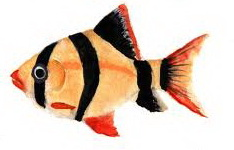#### Interview Puzzles

Stack of 'must-know' interview puzzles !

### Puzzle: How long is the Fish?

2812 views
```Yesterday was a successful fishing day for James. He caught a fairly large golden fish. As he started to measure the fish, he realized that his scale was too short to measure the same.

However he decided to measure in parts(head + body + tail). He somehow measured the Head and found it to be 10cm long. He then measured the Tail to be = (half the Body length + length of Head). If the Body length = (length of Head + length of Tail), help James in calculating the total length of the Fish.```
 1

By  ShaanShaan   5 years ago Fish = Head + Body + Tail Let length of Body = x cm Given : Head = 10 cm Tail = (x/2 + 10) cm Body = (Head + Tail) x = 10 + (x/2 + 10) x/2 = 20 x = 40 cm hence Body = 40 cm So, Tail = 40/2 + 10 = 30 cm Total length of Fish = 10 + 40 + 30 = 80 cmAbhishek Kumar   5 years ago 60cmBrijesh Verma   4 years ago M=h+b+t; :1 t=.5b+h :2 b=h+t :3 soving 2 and 3 t=3h=20 b=2t-2h=40 so M=40+30+10=80Sooraj Dwivedi   8 months ago 30 cmSooraj Dwivedi   8 months ago That is tail length .. total lenght will 30+40+10=80